6470.0.55.001 - Information Paper: Introduction of the 17th Series Australian Consumer Price Index, 2017
Latest ISSUE Released at 11:30 AM (CANBERRA TIME) 06/11/2017  First Issue
Page tools:Print AllRSSSearch this Product

APPENDIX 3 - CALCULATING EC CONTRIBUTIONS TO CHANGE WITH AN ANNUALLY RE–WEIGHTED CPI

1 The availability of annual HFCE data from the National Accounts provides the ABS with an opportunity to update CPI EC level weights more frequently. However, the annual re–weight of the Australian CPI will pose a challenge when calculating contributions to the annual percentage change in the All groups CPI. This is because the contribution will depend not only on changes in prices, but also changes in the weights.

2 To address this, the ABS investigated an approach to estimate the contributions of individual ECs to the annual percentage change of the All groups CPI under annual re–weighting. This methodology was published in Information Paper: An Implementation Plan to Annually Re–weight the Australian CPI (cat. no. 6401.0.60.005).

3 In the current CPI where the weights are fixed for a number of years, deriving the contribution of ECs to the annual rate of inflation does not pose a problem, since the annual rate of inflation is based on a single set of weights. The annual rate of inflation for any given period is derived as the percentage change between the index number in that period, and the index number from the corresponding quarter of the previous year. For example, the annual rate of inflation in March quarter 2019 is the percentage change between the March quarter 2019 and March quarter 2018 index numbers.

4 The proposed approach, stated below, derives an approximate expression for the annual rate of inflation that distinguishes between the contribution due to the percentage price changes of the ECs and changes in the weights of the ECs. For a single EC n, in March quarter 2019, the contribution can be written as: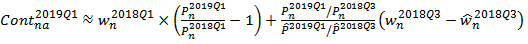where:= weight of EC n to All groups CPI in March quarter 2018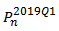= price index of EC n in March quarter 2019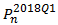= price index of EC n in March quarter 2018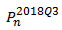= price index of EC n in September quarter 2018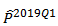= price index of the All groups CPI in March quarter 2019 using the weights of the previous short–term series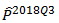= price index of the All groups CPI in September quarter 2018 using the weights of the previous short–term series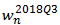= weight of EC n to the All groups CPI in September quarter 2018= weight of EC n to the All groups CPI in September quarter 2018 under the previous short–term series

Detailed information on the decomposition is available from the Appendix of Information Paper: An Implementation Plan to Annually Re–weight the Australian CPI (cat. no. 6401.0.60.005).

5 The ABS has conducted an empirical analysis to assess the proposed methodology, using price indexes compiled from annually updated weights. The t-1 indexes from the Information Paper: Increasing the Frequency of CPI Expenditure Class Weight Updates (cat. no. 6401.0.60.002) formed the basis of this empirical test. As noted in this paper, these indexes span the period September quarter 2005 to September quarter 2015 and exclude the Deposit and loan facilities (direct charges) EC.

6 This proposed method was applied by decomposing the equation into the two components. The first component of the equation measures the contribution of an EC due to its annual percentage change. Summed across all ECs, this component is expected to be slightly higher than the annual inflation rate based on the chained index, as annually updating the weights most likely lowers measured inflation. The empirical results from the analysis support this relationship.

7 The second component measures the contribution of each EC to the annual inflation rate due to the change in its expenditure share, where the expenditure shares are made 'comparable' by price updating them to the link quarter. Summed across all ECs, this component is generally expected to be small and negative. The empirical results from the analysis support this relationship.

8 Lastly, summing the two components across all ECs approximates the annual rate of inflation in the All groups CPI exactly to 2 decimal points over the entire analysis period, demonstrating that the decomposition works well.